InFlight - Updating default data - Page 21 - WoWInterface
11-27-20, 03:02 AM   #401
adekyngt
A Defias Bandit
Join Date: Mar 2019
Posts: 3
SL Updates

Some SL updates
Attached Files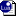InFlight.lua (4.2 KB, 150 views)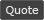11-28-20, 01:37 PM   #402
FoxBJK
A Murloc Raider
Join Date: Oct 2008
Posts: 4
Adding my lua file
Attached FilesInFlight.lua (3.1 KB, 134 views)11-28-20, 05:22 PM   #403
agentdroli
A Kobold Labourer
Join Date: Jul 2018
Posts: 1
some flightpoint for shadowlands
Attached FilesInFlight.lua (2.2 KB, 145 views)11-29-20, 03:32 AM   #404
Norrodar
A Kobold Labourer
Join Date: Nov 2020
Posts: 1
Some new in Azeroth (vanilla), also SL
thanks mate
Attached FilesInFlight.lua (2.5 KB, 139 views)11-30-20, 02:49 PM   #405
lokiloup
A Kobold Labourer
Join Date: Nov 2020
Posts: 1
here ya go from SL
Attached FilesInFlight.lua (2.3 KB, 131 views)

Last edited by lokiloup : 11-30-20 at 02:49 PM. Reason: adding sl11-30-20, 10:28 PM   #406
cborga
A Kobold Labourer
Join Date: Feb 2008
Posts: 1
SL Data

Here my saved variables from Shadowlands.
Attached FilesInFlight.lua (1.6 KB, 112 views)12-01-20, 05:51 AM   #407
gna582
A Kobold Labourer
Join Date: Dec 2020
Posts: 1
thx for developing this. helps me zone out while flying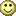Attached FilesInFlight.lua (1.1 KB, 136 views)12-02-20, 03:53 AM   #408
grimrepo941
A Murloc Raider
Join Date: Feb 2019
Posts: 5
Alliance SL flight paths

Contribution since starting SL
Attached FilesInFlight.lua (34.4 KB, 151 views)12-02-20, 07:00 AM   #409
Talaren
A Kobold Labourer
Join Date: May 2009
Posts: 1
fly paths german - Horde + Alliance
Attached FilesInFlight.lua (3.3 KB, 136 views)12-02-20, 08:38 AM   #410
Skawalka
A Defias Bandit
Join Date: Jul 2020
Posts: 2
Flight paths
Attached FilesInFlight.lua (1.9 KB, 127 views)12-06-20, 08:39 PM   #411
SSyl
A Kobold Labourer
Join Date: Dec 2020
Posts: 1
Whole bunch of flight paths all over Shadowlands from random points all over.
Attached FilesInFlight.lua (4.3 KB, 104 views)12-08-20, 09:24 AM   #412
Ereki
A Fallenroot Satyr
Join Date: Apr 2008
Posts: 25
Some SL flights
Attached FilesInFlight.lua (6.4 KB, 108 views)12-08-20, 01:48 PM   #413
LadySilverwolf
Premium Member
InFlight Data

Mostly BFA Horde side, but there may be some alliance smatterings in there too.

Addon mentioned I had a new flight path, so figured I would submit the info.
Attached FilesInFlight.lua (2.7 KB, 94 views)
__________________
~ Strength of Character withstands the Test of Time. ~12-11-20, 10:03 AM   #414
PacFu
A Kobold Labourer
Join Date: Aug 2012
Posts: 1
lua

my upload for inflight timer. I have for a few ingame messages that some of mine are in fact new
Attached FilesInFlight.lua (3.5 KB, 73 views)12-14-20, 03:08 AM   #415
mackay
A Kobold Labourer
Join Date: Dec 2020
Posts: 1
Alliance Shadowlands: I have merged some of the above and included the one I gathered.
In addition i have created a deDE translation file for the germansthey should be correct but not all are included. but better than nothing

translation needst to go here:
_retail_\Interface\AddOns\InFlight_Load\locales
Attached FilesdeDE.lua (3.5 KB, 87 views)InFlight.lua (6.0 KB, 69 views)12-14-20, 06:55 AM   #416
oopinou
A Kobold Labourer
Join Date: Oct 2019
Posts: 1
update horde wow classic
Attached FilesInFlight.lua (1.2 KB, 65 views)12-14-20, 01:47 PM   #417
RavenDT
A Defias Bandit
Join Date: Oct 2006
Posts: 3
Code:
```InFlightDB = {
["version"] = "retail",
["global"] = {
["Alliance"] = {
 = {
 = 59,
["name"] = "Old Gate",
 = 46,
},
 = {
 = 41,
["name"] = "Pridefall Hamlet",
 = 49,
 = 30,
 = 67,
 = 54,
},
 = {
["name"] = "Menagerie of the Master",
 = 51,
},
 = {
 = 38,
["name"] = "Wanecrypt Hill",
 = 46,
 = 64,
 = 30,
},
 = {
["name"] = "Aspirant's Rest",
 = 123,
 = 43,
 = 73,
 = 143,
},
 = {
 = 25,
["name"] = "Sagehaven",
 = 51,
},
 = {
 = 45,
["name"] = "The Spearhead",
 = 29,
},
 = {
["name"] = "Spider's Watch",
 = 56,
},
 = {
 = 39,
 = 40,
["name"] = "Renounced Bastille",
},
 = {
 = 372,
},
 = {
 = 151,
["name"] = "Glitterfall Basin",
 = 232,
 = 28,
 = 37,
},
 = {
["name"] = "Root-Home",
 = 31,
},
 = {
["name"] = "Claw's Edge",
 = 48,
 = 54,
},
 = {
 = 30,
["name"] = "Refugee Camp",
 = 40,
 = 30,
},
 = {
 = 62,
},
 = {
 = 118,
},
 = {
 = 8,
 = 8,
["name"] = "Elysian Hold",
 = 8,
},
 = {
 = 8,
["name"] = "Hero's Rest",
 = 4,
},
 = {
 = 4,
 = 8,
["name"] = "Aspirant's Rest",
 = 3,
},
 = {
 = 4,
 = 8,
["name"] = "Xandria's Vigil",
 = 4,
},
 = {
 = 24,
 = 64,
["name"] = "Terrace of the Collectors",
},
 = {
 = 189,
},
 = {
 = 17,
["name"] = "Charred Ramparts",
 = 188,
 = 46,
 = 44,
},
 = {
 = 42,
 = 261,
["name"] = "Sanctuary of the Mad",
},
 = {
 = 33,
["name"] = "Theater of Pain",
 = 77,
},
 = {
 = 54,
["name"] = "Dominance Keep",
 = 29,
},
 = {
 = 293,
 = 161,
},
 = {
 = 56,
 = 40,
 = 28,
 = 26,
["name"] = "Tirna Vaal",
},
 = {
 = 73,
},
 = {
 = 91,
 = 92,
 = 129,
 = 103,
 = 87,
 = 125,
 = 105,
 = 128,
 = 81,
 = 70,
 = 138,
 = 79,
 = 98,
 = 76,
 = 117,
["name"] = "Oribos",
 = 55,
 = 91,
 = 63,
 = 144,
},
 = {
["name"] = "Bleak Redoubt",
 = 18,
},
 = {
 = 120,
},
 = {
 = 316,
},
 = {
 = 87,
["name"] = "Elysian Hold",
 = 222,
 = 224,
 = 53,
 = 50,
},
 = {
 = 29,
 = 30,
["name"] = "Darkhaven",
},
 = {
 = 47,
["name"] = "Hero's Rest",
 = 44,
},
},
},
["upload"] = 1608606647,
["dbinit"] = 827,
}```12-14-20, 11:18 PM   #418
davep1553
A Kobold Labourer
Join Date: Apr 2011
Posts: 1
SL Updates

Our Two Files

Hope you can get an update out with all the data soon. Happy Holidays.
Attached FilesInFlight.lua (4.2 KB, 71 views)InFlight2.lua (2.3 KB, 60 views)

Last edited by davep1553 : 12-14-20 at 11:20 PM.12-18-20, 12:05 PM   #419
adekyngt
A Defias Bandit
Join Date: Mar 2019
Posts: 3
More updates

Moar data for the timer.
Attached FilesInFlight.lua (6.3 KB, 54 views)12-18-20, 01:02 PM   #420
endradon
A Kobold Labourer
Join Date: Dec 2020
Posts: 1
Some SL FPs (with Kyrian Gateways)

Lua Code:
`InFlightDB = {    ["version"] = "retail",    ["global"] = {        ["Alliance"] = {             = {                ["name"] = "Sanctuary of the Mad",                 = 43,            },             = {                ["name"] = "Old Gate",                 = 47,            },             = {                ["name"] = "Pridefall Hamlet",                 = 40,            },             = {                ["name"] = "Menagerie of the Master",                 = 51,            },             = {                ["name"] = "Wanecrypt Hill",                 = 44,            },             = {                 = 71,                 = 43,                ["name"] = "Aspirant's Rest",                 = 173,            },             = {                 = 153,                 = 53,                ["name"] = "Elysian Hold",                 = 232,            },             = {                 = 133,            },             = {                ["name"] = "The Spearhead",                 = 29,            },             = {                ["name"] = "Keres' Rest",                 = 38,            },             = {                ["name"] = "Renounced Bastille",                 = 35,            },             = {                 = 57,                ["name"] = "Glitterfall Basin",                 = 37,            },             = {                ["name"] = "Root-Home",                 = 61,            },             = {                ["name"] = "Claw's Edge",                 = 55,            },             = {                ["name"] = "Refugee Camp",                 = 30,            },             = {                 = 4,                 = 7,                ["name"] = "Hero's Rest",                 = 4,            },             = {                 = 4,                 = 4,                ["name"] = "Aspirant's Rest",                 = 7,            },             = {                 = 4,                 = 7,                ["name"] = "Xandria's Vigil",                 = 4,            },             = {                ["name"] = "Terrace of the Collectors",                 = 56,            },             = {                 = 137,            },             = {                 = 109,            },             = {                 = 155,            },             = {                 = 73,                 = 156,            },             = {                 = 32,            },             = {                 = 29,            },             = {                 = 23,                 = 32,            },             = {                ["name"] = "Hibernal Hollow",                 = 26,            },             = {                 = 27,                 = 47,                ["name"] = "Hero's Rest",            },             = {                 = 143,            },             = {                 = 90,            },             = {                 = 55,                 = 117,                 = 104,                 = 102,                 = 71,                 = 91,                 = 92,                 = 78,                 = 103,                 = 80,                 = 105,                ["name"] = "Oribos",            },             = {                 = 28,                ["name"] = "Tirna Vaal",                 = 67,            },             = {                 = 7,                 = 7,                ["name"] = "Elysian Hold",                 = 7,            },             = {                 = 35,                 = 32,                ["name"] = "Darkhaven",            },             = {                ["name"] = "Dominance Keep",                 = 77,            },             = {                 = 141,            },             = {                 = 178,                 = 44,                ["name"] = "Theater of Pain",            },        },    },    ["upload"] = 1609241885,    ["dbinit"] = 827,}`WoWInterface » InFlight - Updating default data

 Thread Tools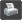Show Printable Version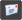Email this Page Display Modes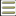Linear Mode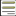Switch to Hybrid Mode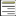Switch to Threaded Mode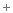Posting Rules You may not post new threads You may not post replies You may not post attachments You may not edit your posts vB code is On Smilies are On [IMG] code is On HTML code is Off